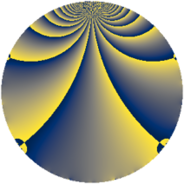# Properties

 Label 2366.2.mLevel $2366$ Weight $2$ Character orbit 2366.m Rep. character $\chi_{2366}(1037,\cdot)$ Character field $\Q(\zeta_{6})$ Dimension $152$ Sturm bound $728$

# Related objects

## Defining parameters

 Level: $$N$$ $$=$$ $$2366 = 2 \cdot 7 \cdot 13^{2}$$ Weight: $$k$$ $$=$$ $$2$$ Character orbit: $$[\chi]$$ $$=$$ 2366.m (of order $$6$$ and degree $$2$$) Character conductor: $$\operatorname{cond}(\chi)$$ $$=$$ $$13$$ Character field: $$\Q(\zeta_{6})$$ Sturm bound: $$728$$

## Dimensions

The following table gives the dimensions of various subspaces of $$M_{2}(2366, [\chi])$$.

Total New Old
Modular forms 784 152 632
Cusp forms 672 152 520
Eisenstein series 112 0 112

## Trace form

 $$152q + 76q^{4} - 76q^{9} + O(q^{10})$$ $$152q + 76q^{4} - 76q^{9} + 4q^{10} + 12q^{11} - 8q^{14} + 12q^{15} - 76q^{16} + 4q^{17} + 4q^{22} + 4q^{23} - 168q^{25} - 24q^{27} - 16q^{30} - 24q^{33} - 4q^{35} + 76q^{36} - 12q^{37} - 16q^{38} + 8q^{40} + 36q^{41} + 4q^{42} - 8q^{43} - 60q^{45} + 12q^{46} + 76q^{49} + 12q^{50} + 40q^{51} - 48q^{53} + 36q^{54} + 4q^{55} - 4q^{56} - 24q^{58} + 8q^{61} - 12q^{62} + 12q^{63} - 152q^{64} + 48q^{66} + 12q^{67} - 4q^{68} - 4q^{69} + 12q^{72} - 16q^{74} + 40q^{75} + 16q^{79} - 64q^{81} - 12q^{84} - 60q^{85} - 4q^{87} - 4q^{88} - 48q^{90} + 8q^{92} - 48q^{93} - 24q^{94} - 28q^{95} - 48q^{97} + O(q^{100})$$

## Decomposition of $$S_{2}^{\mathrm{new}}(2366, [\chi])$$ into newform subspaces

The newforms in this space have not yet been added to the LMFDB.

## Decomposition of $$S_{2}^{\mathrm{old}}(2366, [\chi])$$ into lower level spaces

$$S_{2}^{\mathrm{old}}(2366, [\chi]) \cong$$ $$S_{2}^{\mathrm{new}}(13, [\chi])$$$$^{\oplus 8}$$$$\oplus$$$$S_{2}^{\mathrm{new}}(91, [\chi])$$$$^{\oplus 4}$$$$\oplus$$$$S_{2}^{\mathrm{new}}(169, [\chi])$$$$^{\oplus 4}$$$$\oplus$$$$S_{2}^{\mathrm{new}}(182, [\chi])$$$$^{\oplus 2}$$$$\oplus$$$$S_{2}^{\mathrm{new}}(338, [\chi])$$$$^{\oplus 2}$$$$\oplus$$$$S_{2}^{\mathrm{new}}(1183, [\chi])$$$$^{\oplus 2}$$